Himanshi Singh — Published On March 22, 2021 and Last Modified On March 30th, 2021

## Introduction

In the previous article- How to Split a Decision Tree – The Pursuit to Achieve Pure Nodes, you understood the basics of Decision Trees such as splitting, ideal split, and pure nodes. In this article, we’ll see one of the most popular algorithms for selecting the best split in decision trees- Gini Impurity.

Note: If you are more interested in learning concepts in an Audio-Visual format, We have this entire article explained in the video below. If not, you may continue reading.

P.S – If you haven’t read previous the article then there are chances you might face difficulties in understanding this article.

So, till now we’ve seen that the attribute “Class” is able to estimate the student’s behavior, about playing cricket or not. And this attribute is doing a much better job compared to the remaining two variables, like “the height” and “the performance in the class”. If you recall we made a split on all the available features and then compare each split to decide which one was the best. That is how the decision tree algorithm also works.

A Decision Tree first splits the nodes on all the available variables and then selects the split which results in the most homogeneous sub-nodes.

Homogeneous here means having similar behavior with respect to the problem that we have. If the nodes are entirely pure, each node will only contain a single class and hence they will be homogeneous. So intuitively you can imagine that the more the purity of the nodes more will be the homogeneity.

## Gini impurity: A Decision tree algorithm for selecting the best split

There are multiple algorithms that are used by the decision tree to decide the best split for the problem. Let’s first look at the most common and popular out of all of them, which is Gini Impurity. It measures the impurity of the nodes and is calculated as:Let’s first understand what Gini is and then I’ll show you how you can calculate the Gini impurity for split and decide the right split. Let’s say we have a node like this-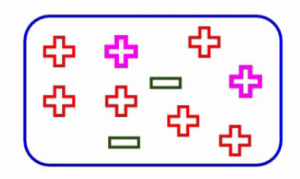So what Gini says is that if we pick two points from a population at random, the pink ones highlighted here, then they must be from the same class. Let’s say we have a completely pure node-Can you guess what would be the probability that a randomly picked point will belong to the same class? Well, obviously it will be 1 since all the points here belong to the same class. So no matter which two points you picked, they will always belong to that one class and hence the probability will always be 1 if the node is pure. And that is what we want to achieve using Gini.

Gini ranges from zero to one, as it is a probability and the higher this value, the more will be the purity of the nodes. And of course, a lesser value means lesser pure nodes.

## Properties of Gini impurity

Let’s look at its properties before we actually calculate the Gini impurity to decide the best split.

We decide the best split based on the Gini impurity and as we discussed before, Gini impurity is:Here Gini denotes the purity and hence Gini impurity tells us about the impurity of nodes. Lower the Gini impurity we can safely infer the purity will be more and hence a higher chance of the homogeneity of the nodes.

Gini works only in those scenarios where we have categorical targets. It does not work with continuous targets.

A very important point to note to keep in mind. For example, if you want to predict the house price or the number of bikes that have been rented, Gini is not the right algorithm. It only performs binary splits either yes or no, success or failure, and so on. So it will only split a node into two sub-nodes. These are the properties of Gini impurity.

## Steps to calculate Gini impurity for a Split

Let’s now look at the steps to calculate the Gini split. First, we calculate the Gini impurity for sub-nodes, as you’ve already discussed Gini impurity is, and I’m sure you know this by now:

#### Gini impurity =  1 –  Gini

Here is the sum of squares of success probabilities of each class and is given as: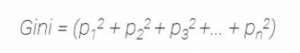Considering that there are n classes.

Once we’ve calculated the Gini impurity for sub-nodes, we calculate the Gini impurity of the split using the weighted impurity of both sub-nodes of that split. Here the weight is decided by the number of observations of samples in both the nodes. Let’s look at these calculations using an example, which will help you understand this even better.

For the split on the performance in the class, remember this is how the split was?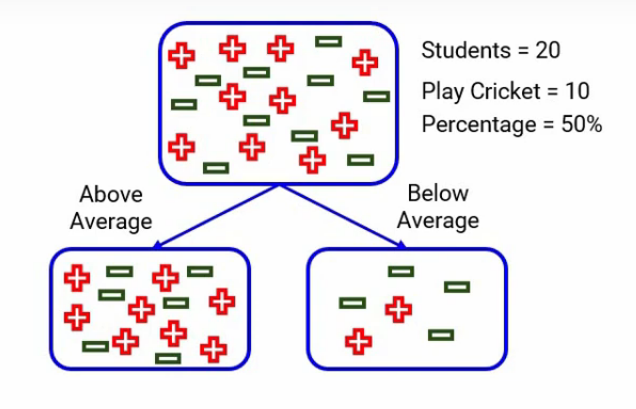Split on Performance in Class

We have two categories, one is “above average” and the other one was “below average”. When we focus on the above average, we have 14 students out of which 8 play cricket and 6 do not. The probability of playing cricket would be 8 divided by 14, which is around 0.57, and similarly, for not playing cricket, the probability will be 6 divided by 14, which will be around 0.43. Here for simplicity, I’ve rounded up the calculations rather than taking the exact number.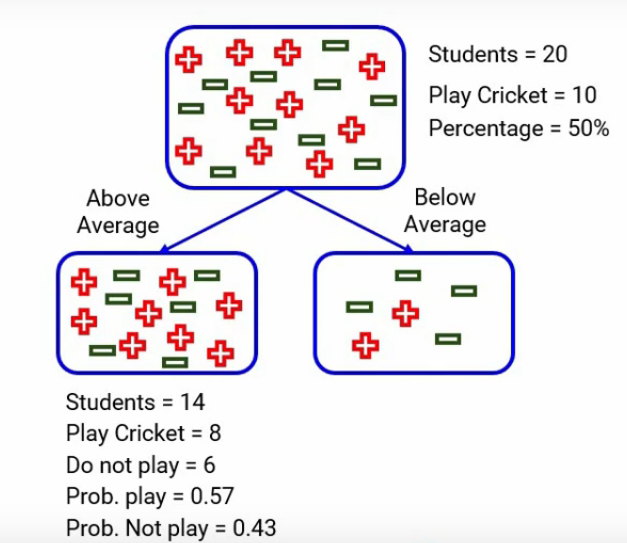Similarly, when we look at below average, we calculated all the numbers and here they are- the probability of playing is 0.33 and of not playing is 0.67-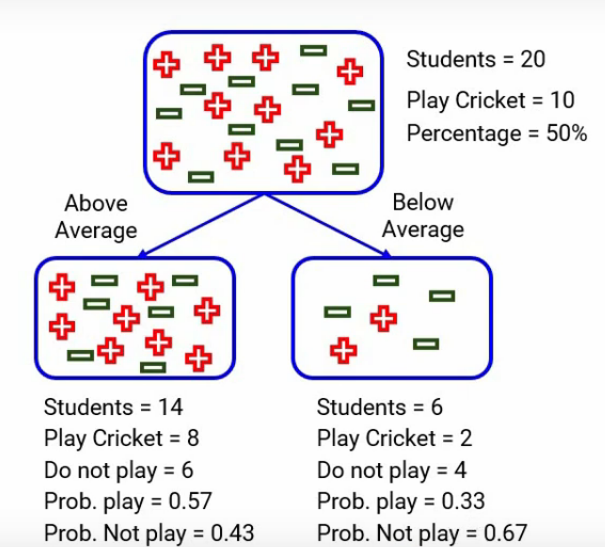Let’s now calculate the Gini impurity of the sub-nodes for above average and here’s the calculation-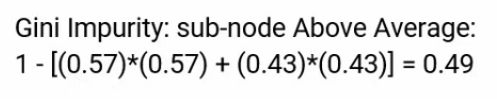It will be, one minus the square of the probability of success for each category, which is 0.57 for playing cricket and 0.43 for not playing cricket. So after this calculation Gini comes out to be around 0.49. The Below average node will do the same calculation as Gini. For below average: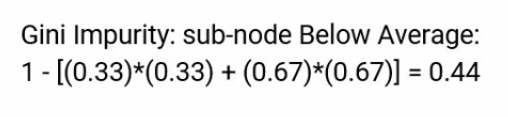It comes up to be around 0.44. Just take a pause and analyze these numbers.

Now to calculate the Gini impurity of the split, we will take the weighted Gini impurities of both nodes, above average and below average. In this case, the weight of a node is the number of samples in that node divided by the total number of samples in the parent node. So for the above-average node here, the weight will be 14/20, as there are 14 students who performed above the average of the total 20 students that we had.

And the weight for below average is 6/20. So, the weighted Gini impurity will be the weight of that node multiplied by the Gini impurity of that node. The weighted Gini impurity for performance in class split comes out to be: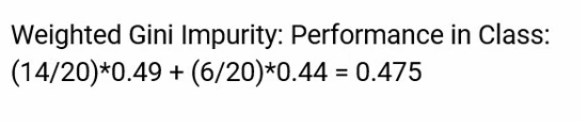Similarly, here we have captured the Gini impurity for the split on class, which comes out to be around 0.32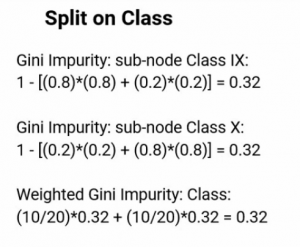Now, if we compare the two Gini impurities for each split-We see that the Gini impurity for the split on Class is less. And hence class will be the first split of this decision tree.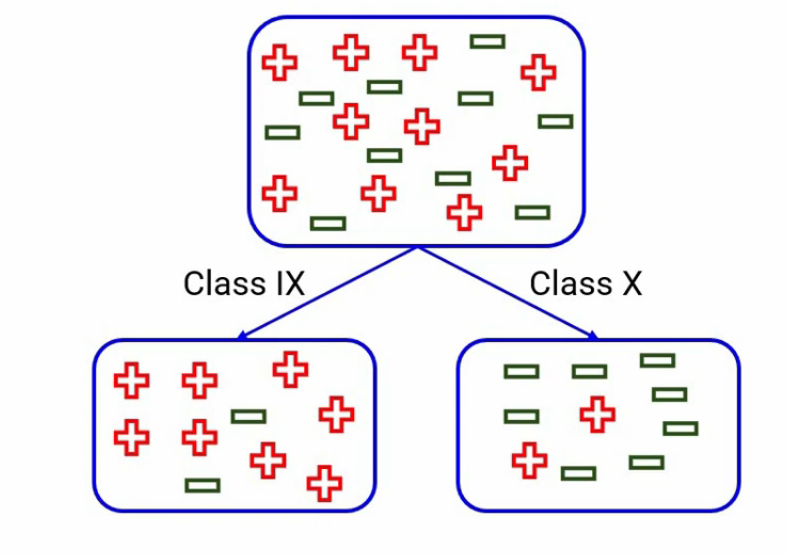Split on Class

Similarly, for each split, we will calculate the Gini impurities and the split producing minimum Gini impurity will be selected as the split. And you know, that the minimum value of Gini impurity means that the node will be purer and more homogeneous.

## End Notes

In this article, we saw one of the most popular splitting algorithms in Decision Trees- Gini Impurity. It can be used for categorical target variables only. There are other algorithms as well used for splitting, which if you want to understand you can let me know in the comment section.

If you are looking to kick start your Data Science Journey and want every topic under one roof, your search stops here. Check out Analytics Vidhya’s Certified AI & ML BlackBelt Plus Program

If you have any questions, let me know in the comments section!###### Himanshi Singh

I am a data lover and I love to extract and understand the hidden patterns in the data. I want to learn and grow in the field of Machine Learning and Data Science.

## 9 thoughts on "How to select Best Split in Decision trees using Gini Impurity"###### Anurag Singh says:March 22, 2021 at 7:18 pm###### Himanshi Singh says:March 22, 2021 at 9:05 pm###### Lê Quang Khánh says:March 23, 2021 at 8:58 am###### Himanshi Singh says:March 23, 2021 at 10:06 am###### Maak says:May 20, 2021 at 11:08 am